## Recurrent Neural Networks in SAS Viya

Started ‎05-24-2023 by
Modified ‎05-24-2023 by
Views 293

RNNS are useful in working with any data where order matters. Examples include:

• Natural Language Understanding (NLU) and Natural Language Generation (NLG), for example “Beth drank coffee” is not the same as “Coffee drank Beth.”
• Forecasting, for example, forecasting profits
• Physical processes, for example, erosion
• Image captioning, where the input is an image, and the output is text

This blog will provide a little background, describe the ways you can create RNNs in SAS Viya, and provide example code.

RNNs are called recurrent because the network feeds back on itself. RNNs are built with Long Short-Term Memory (LSTM) models or gated recurrent units (GRUs)  One advantage of LSTM models is that they can “remember” dependencies for long periods of time.

This process is explained in detail in an excellent 30-minute video by Brandon Rohrer. If you have time, watch the video. If not, the next 3 images provide a quick synopsis.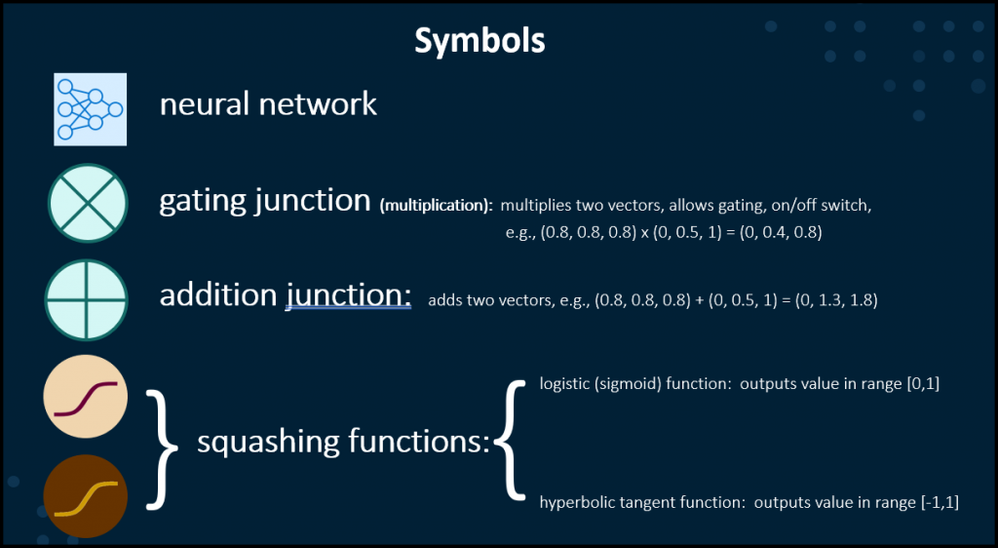A recurrent neural network starts with new information which runs through a neural network. Then a “squashing” function (activation function, link function) is used to map the output of a neuron to a limited range. I don’t know who first coined the term squashing function, but I love it. Very descriptive. You are essentially taking any real number and squasing it down to a specific limited range. Commonly used squashing functions are:

• Sigmoid function, which maps the output to the range of 0 to 1Select any image to see a larger version.
Mobile users: To view the images, select the "Full" version at the bottom of the page.

• Hyperbolic tangent, which maps the output to a range of -1 to 1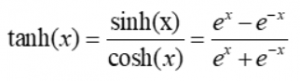Both of these functions are used in the full RNN LSTM process. Hyperbolic tangent is commonly used to squash the output from a neural network to a number from -1 to 1. This number from -1 to 1 is now your prediction and can also be fed back into the neural network again as shown below.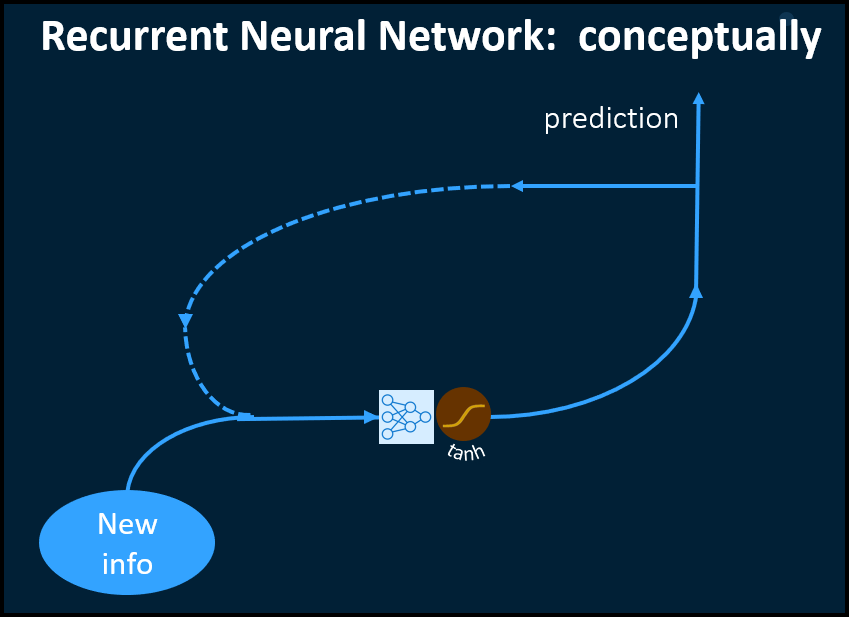A recurrent neural network uses long short-term memory process to make decision in multiple steps. The essential function for making these decisions (selection, forgetting, and ignoring) is a gate. Mathematically, the gate is simple a multiplication function where the output of the previous step is multiplied by a number. Multiplication times zero is a closed gate; that output is stopped right there. Multiplication by 1 is a fully open gate; the output advances fully intact. You can also have a halfway open gate by multiplying by 0.5, and so on.

An RNN with LSTM has:

• PREDICTION BRANCH: From new input information, an neural makes a prediction squashed between [-1,1] by a hyperbolic tangent function, then passed on to the next phase
• IGNORING BRANCH: Sets aside irrelevant information, so it doesn’t affect the other predictions.
• FORGETTING BRANCH: Adds the predictions to the information coming from the first gate. The neural network and the squashing function decide what information will be forgotten and what information will be kept. Then the multiplication junction (gating) determines how much of the information is going to pass to the next phase.
• SELECTION BRANCH: Both new information and previous information are inputs. This neural network selects which memory and collected possibilities will be considered to make new predictions.

This is illustrated conceptually below: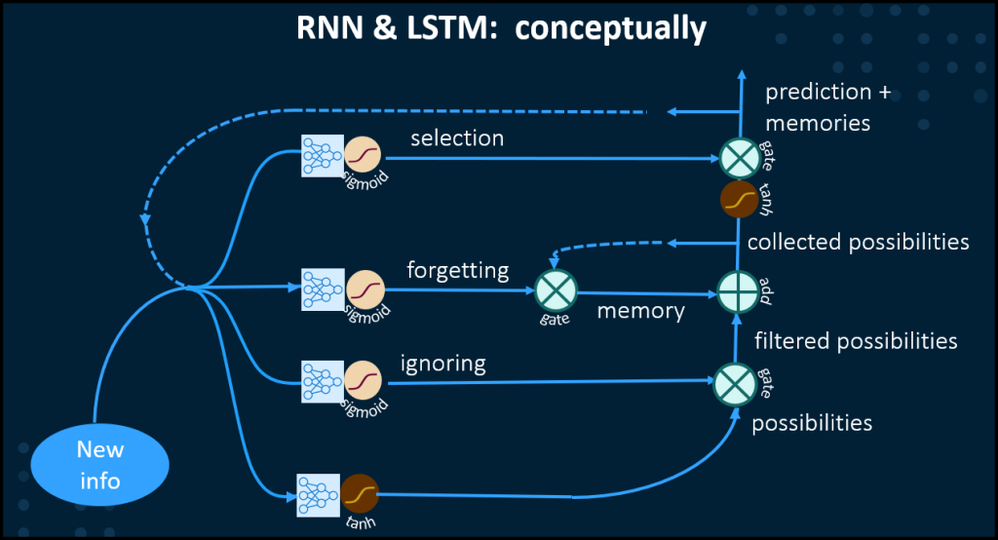RNNs in SAS Viya

SAS Viya has two CAS action sets that allow you to build, train, score, and export RNNs.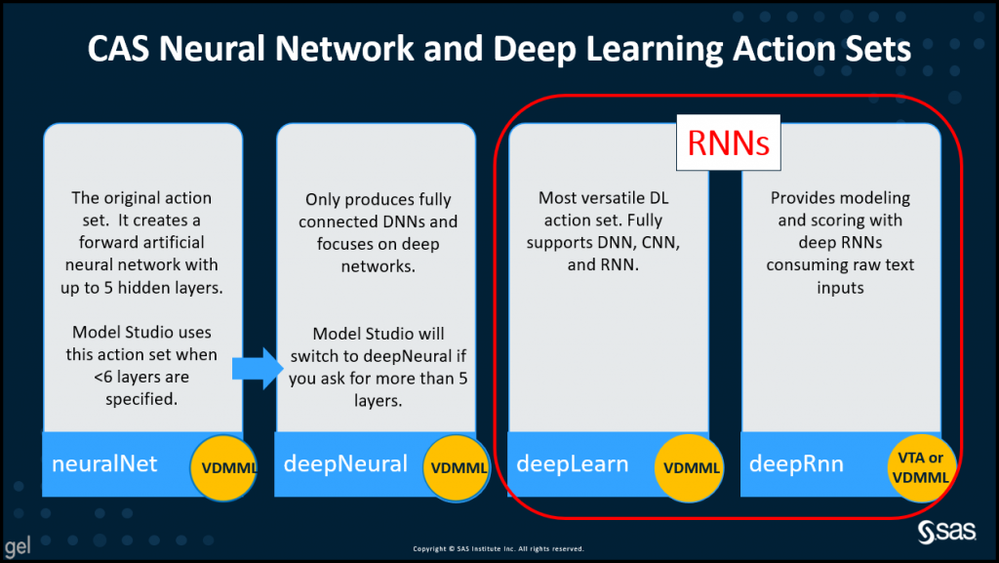The deepRNN action set is designed specifically for RNNs. It uses one of two loss functions:

• For classification, an entropy loss function
• For regression, normal loss function

The deepRNN action set includes 3 actions as shown below.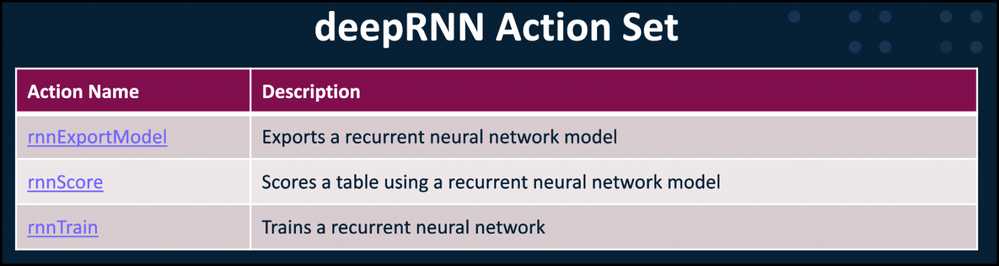The deepLearn action set is highly flexible and lets you build many types of neural networks. With respect to RNNS, the deepLearn action set supports both long short-term memory (LSTM) and gated recurrent unit (GRU) RNNs.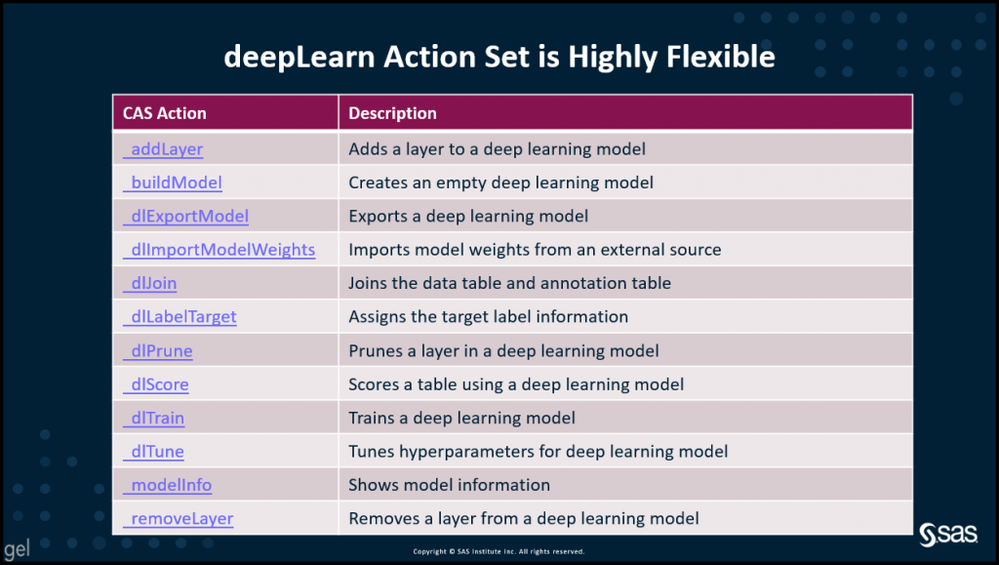RNN Coding in Python Using SWAT to Run CAS Actions

To build your own RNN layer by layer using the deepLearn action set, you would first start a session and load the action set.

``````sess = swat.CAS('sas-cas-server-default-bin', portnumber, 'gatedemoXXX', 'lnxsas', caslib="casuser")

Then you would build an LSTM model (here the model is named classifer)

``````sess.buildmodel(model=dict(name='classifier',
replace=True
),
type='RNN'
)``````

``````sess.addlayer(model='classifier',
name='data',
layer=dict(type='input')
)``````

``````sess.addlayer(model='classifier',
name='rnn11',
srclayers=['data'],
layer=dict(type='recurrent',
n=50,
init='xavier',
rnnType='LSTM',
act='TANH',
outputType='samelength',
reverse=False
)
)

name='rnn21',
srclayers=['rnn11'],
layer=dict(type='recurrent',
n=50,
init='xavier',
rnnType='LSTM',
act='TANH',
outputType='samelength',
reverse=False
)
)

name='rnn31',
srclayers=['rnn21'],
layer=dict(type='recurrent',
n=50,
init='xavier',
rnnType='LSTM',
act='TANH',
outputType='encoding',
reverse=False
)
)
``````

``````sess.addlayer(model='classifier',
name='outlayer',
srclayers=['fc1'],
layer=dict(type='output',
act='SOFTMAX'
)
)``````

(The above code is from SAS Viya documentation )

Recall that the softmax function takes a vector of scores and transforms it to a vector of values between 0 and 1 that sum to 1.For example: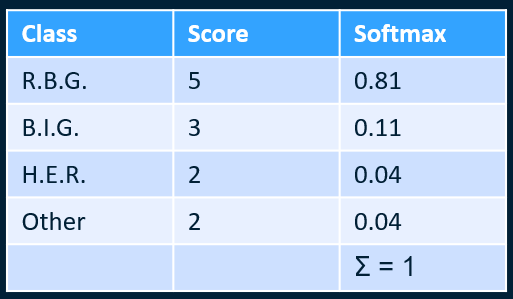RNNs on GPUs

SAS supports using GPUs for recurrent neural networks to reduce processing time, but keep in mind that the underlying algorithm is slightly different when run on GPUs than when on CPUs

There are a number of requirements, including:

• The RNN can have only a single input layer and a single output layer
• Recurrent layers must be either unidirectional forward layers or bidirectional layers
• All recurrent layers must use the same memory cell type (LSTM, GRU, RNN, or PASSTHROUGH)
• All recurrent layers must have the same hidden dimension
• Text generation with RNNs on GPUs is not supported

Dilated RNNs Available via SAS Viya dlModelZoo

SAS Viya dlModelZoo provides a variety of predefined PyTorch models that can be used OOTB, including dilated RNN. Dilated Rnns are designed to help address RNN training issues, such as complex dependencies, vanishing gradients, and exploding gradients. Dilated RNNs are also easily parallelized, and may require fewer parameters.